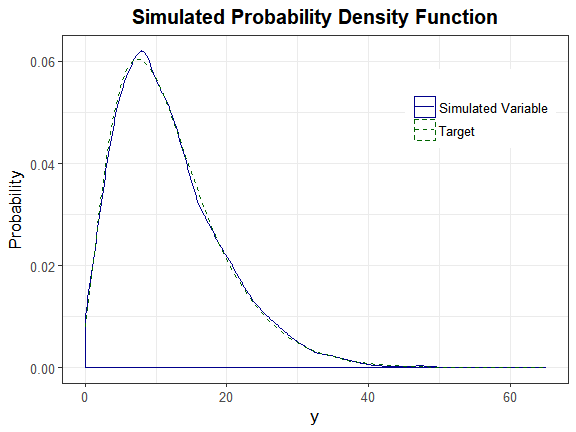# Overall Workflow for Data Simulation

#### 2018-06-28

A step-by-step guideline for data simulation is as follows:

1. Obtain the distribution parameters for the desired variables.

1. Continuous variables: these are mean, variance, skewness and standardized kurtosis (kurtosis - 3) for Fleishman (1978)’s third-order method, plus standardized fifth and sixth cumulants for Headrick (2002)’s fifth-order method. If the goal is to simulate a theoretical distribution (i.e. Gamma, Beta, Logistic, etc.), these values can be obtained using calc_theory. If the goal is to mimic an empirical data set, these values can be found using calc_moments (using the method of moments) or calc_fisherk (using Fisher’s k-statistics). If the standardized cumulants are obtained from calc_theory, the user may need to use rounded values as inputs (i.e. skews = round(skews, 8)). Due to the nature of the integration involved in calc_theory, the results are approximations. Greater accuracy can be achieved by increasing the number of subdivisions (sub) used in the integration process. For example, in order to ensure that skew is exactly 0 for symmetric distributions. For some sets of cumulants, it is either not possible to find power method constants or the calculated constants do not generate valid power method pdfs. In these situations, adding a value to the sixth cumulant may provide solutions (see find_constants). If simulation results indicate that a continuous variable does not generate a valid pdf, the user can try find_constants with various sixth cumulant correction vectors to determine if a valid pdf can be found.
2. Ordinal variables ($$\Large r \ge 2$$ categories): these are the cumulative marginal probabilities and support values (if desired). The probabilities should be combined into a list of length equal to the number of ordinal variables. The i-th element is a vector of the cumulative probabilities defining the marginal distribution of the i-th variable. If the variable can take r values, the vector will contain r - 1 probabilities (the r-th is assumed to be 1). For binary variables, the probability should be the probability of achieving the $$\Large 1^{st}$$ support value. The support values should be combined into a separate list. The i-th element is a vector containing the r ordered support values. If not provided, the default is for the i-th element to be the vector 1, …, r.
3. Poisson variables: the lambda (mean) values should be given as a vector (see stats::dpois).
4. Negative Binomial variables: the sizes (target number of successes) and either the success probabilities or the means should be given as vectors (see see stats::dnbinom). The variable represents the number of failures which occur in a sequence of Bernoulli trials before the target number of successes is achieved.
2. If continuous variables are desired, verify that the standardized kurtoses are greater than the lower kurtosis bounds. These bounds can be calculated using calc_lower_skurt, given the skewness (for method = “Fleishman”) and standardized fifth and sixth cumulants (for method = “Polynomial”, referring to Headrick’s method) for each variable. Different seeds should be examined to see if a lower boundary can be found. If a lower bound produces power method constants that yield an invalid pdf, the user may wish to provide a Skurt vector of kurtosis corrections. In this case, calc_lower_skurt will attempt to find the smallest value that produces a kurtosis which yields a valid power method pdf. In addition, if method = “Polynomial”, a sixth cumulant correction vector (Six) may be used to facilitate convergence of the root-solving algorithm. Since this step can take considerable computation time, the user may instead wish to perform this check after simulation if any of the variables have invalid power method pdfs.

3. Check if the target correlation matrix falls within the feasible correlation bounds, given the parameters for the desired distributions. The ordering of the variables in the correlation matrix MUST be 1st ordinal, 2nd continuous, 3rd Poisson, and 4th Negative Binomial. These bounds can be calculated using either valid_corr (correlation method 1) or valid_corr2 (correlation method 2). Note that falling within these bounds does not guarantee that the target correlation can be achieved. However, the check can alert the user to pairwise correlations that obviously fall outside the bounds.

4. Generate the variables using either correlation method 1 and rcorrvar or correlation method 2 and rcorrvar2. The user may want to try both to see which gives a better approximation to the variables and correlation matrix. The accuracy and simulation time will vary by situation. Again, the ordering of the variables in the correlation matrix MUST be 1st ordinal, 2nd continuous, 3rd Poisson, and 4th Negative Binomial. In addition, the error loop can minimize the correlation errors in most situations.

5. Summarize the results numerically. The functions rcorrvar and rcorrvar2 provide data.frames containing summaries by variable type and the maximum error between the final and target correlation matrices. Additional summary functions include: sim_cdf_prob (to calculate a cumulative probability up to a given continuous y value), power_norm_corr (to calculate the correlation between a continuous variable and the generating standard normal variable), stats_pdf (to calculate the 100 * alpha percent symmetric trimmed mean, median, mode, and maximum height of a valid power method pdf).

6. Summarize the results graphically. Comparing the simulated data to the target distribution demonstrates simulation accuracy. The graphing functions provided in this package can be used to display simulated data values, pdfs, or cdfs. The target distributions (either by theoretical distribution name or given an empirical data set) can be added to the data value or pdf plots. Cumulative probabilities can be added to the cdf plots (for continuous variables).

## Example

The following example generates 3 continuous, 1 binary, 1 ordinal, 3 Poisson, and 2 Negative Binomial variables. The standardized cumulants produce power method constants that yield valid pdfs, so no sixth cumulant corrections are needed. See the Using the Sixth Cumulant Correction to Find Valid Power Method Pdfs vignette for examples of using the sixth cumulant correction. (Note that the printr Xie (2017) package is invoked to display the tables.)

The continuous variables come from the following distributions:

1. Normal($$\Large 0, 1$$)
2. Chisq ($$\Large df = 4$$)
3. Beta ($$\Large \alpha = 4, \beta = 2$$)

The ordinal variables have the following cumulative probabilities:

1. c(0.3, 0.75) (3 categories)
2. c(0.2, 0.5, 0.9) (4 categories)

The last probability in each case is assumed to be 1, and should not be included.

The Poisson variables have the following lambda (mean, lam) values:

1. 1
2. 5
3. 10

The Negative Binomial variables have the following sizes and success probabilities:

1. size <- 3, prob <- 0.2
2. size <- 6, prob <- 0.8

Either success probabilities (prob) or means (mu) should be given for all variables.

### Step 1: Set up the distributions and obtain the standardized cumulants

library("SimMultiCorrData")
library("printr")

# Turn off scientific notation
options(scipen = 999)

# Set seed and sample size
seed <- 11
n <- 10000

# Continuous Distributions
Dist <- c("Gaussian", "Chisq", "Beta")

# Calculate standardized cumulants
# Those for the normal distribution are rounded to ensure the correct values
# are obtained.
M1 <- round(calc_theory(Dist = "Gaussian", params = c(0, 1)), 8)
M2 <- calc_theory(Dist = "Chisq", params = 4)
M3 <- calc_theory(Dist = "Beta", params = c(4, 2))
M <- cbind(M1, M2, M3)

# Binary and Ordinal Distributions
marginal <- list(c(0.3, 0.75), c(0.2, 0.5, 0.9))
support <- list() # default support will be generated inside simulation

# Poisson Distributions
lam <- c(1, 5, 10)

# Negative Binomial Distributions
size <- c(3, 6)
prob <- c(0.2, 0.8)

ncat <- length(marginal)
ncont <- ncol(M)
npois <- length(lam)
nnb <- length(size)

# Create correlation matrix from a uniform distribution (0.2, 0.7)
set.seed(seed)
Rey <- diag(1, nrow = (ncat + ncont + npois + nnb))
for (i in 1:nrow(Rey)) {
for (j in 1:ncol(Rey)) {
if (i > j) Rey[i, j] <- runif(1, 0.2, 0.7)
Rey[j, i] <- Rey[i, j]
}
}

# Check to see if Rey is positive-definite
min(eigen(Rey, symmetric = TRUE)$values) < 0 ##  FALSE ### Step 2: Calculate the lower kurtosis bounds for the continuous variables Since this step takes considerable computation time, the user may wish to calculate these after simulation. Lower <- list() # list of standardized kurtosis values to add in case only invalid power # method pdfs are produced Skurt <- list(seq(0.5, 2, 0.5), seq(0.02, 0.05, 0.01), seq(0.02, 0.05, 0.01)) start.time <- Sys.time() for (i in 1:ncol(M)) { Lower[[i]] <- calc_lower_skurt(method = "Polynomial", skews = M[3, i], fifths = M[5, i], sixths = M[6, i], Skurt = Skurt[[i]], seed = 104) } ## Only invalid pdf constants could be found. ## Try more starting values (increase n) ## or a different seed or Skurt vector. ## Also verify standardized cumulant values. stop.time <- Sys.time() Time <- round(difftime(stop.time, start.time, units = "min"), 3) cat("Total computation time:", Time, "minutes \n") ## Total computation time: 0.703 minutes # Note the message given for Distribution 1 (Normal). In each case, the lower kurtosis boundary calculated from the original Lagrangean constraint equations (see poly_skurt_check) generates constants that yield an invalid power method pdf. This is indicated by the fact that each Invalid.C data.frame contains solutions (i.e. see Lower[]$Invalid.C).

For Distributions 2 and 3, lower kurtosis values that generate constants that yield valid power method pdfs could be found by adding the values displayed in SkurtCorr1 to the original lower kurtosis boundary. For Distribution 1 (Normal), no kurtosis addition (of those specified in Skurt) generated constants that yield a valid pdf. This does not cause a problem since the simulated variable has a valid power method pdf.

Look at lower kurtosis boundaries and sixth cumulant corrections:

1. Normal($$\Large 0,1$$) Distribution:
as.matrix(Lower[]$Min[1, c("skew", "fifth", "sixth", "valid.pdf", "skurtosis")], nrow = 1, ncol = 5, byrow = TRUE)  skew fifth sixth valid.pdf skurtosis 7 0 0 0 FALSE -0.2829743 Note that valid.pdf = FALSE, which means that a kurtosis correction could not be found that yielded constants that produce a valid power method pdf. The original lower kurtosis boundary (see Lower[]$Min) is -0.282974. The standardized kurtosis for the distribution (0) falls above this boundary.

1. Chisq($$\Large df = 4$$) Distribution:
as.matrix(Lower[]$Min[1, c("skew", "fifth", "sixth", "valid.pdf", "skurtosis")], nrow = 1, ncol = 5, byrow = TRUE)  skew fifth sixth valid.pdf skurtosis 1.414214 8.485281 30 TRUE 3.005959 Lower[]$SkurtCorr1
##  0.03

The original lower kurtosis boundary (see Lower[]$Invalid.C) of 2.975959 has been increased to 3.005959, so that the kurtosis correction is 0.03. The standardized kurtosis for the distribution (3) is approximately equal to this boundary. This does not cause a problem since the simulated variable has a valid power method pdf. 1. Beta($$\Large \alpha = 4, \beta = 2$$) Distribution: as.matrix(Lower[]$Min[1, c("skew", "fifth", "sixth", "valid.pdf",
"skurtosis")],
nrow = 1, ncol = 5, byrow = TRUE) 
skew fifth sixth valid.pdf skurtosis
-0.4677072 1.403122 -0.4261364 TRUE -0.3722661
Lower[]$SkurtCorr1 ##  0.02 The original lower kurtosis boundary (see Lower[]$Invalid.C) of -0.392266 has been increased to -0.372266, so that the kurtosis correction is 0.02. The standardized kurtosis for the distribution (-0.2727) falls above this boundary.

The remaining steps vary by simulation method:

### Correlation Method 1

#### Step 3: Verify the target correlation matrix falls within the feasible correlation bounds

# Make sure Rey is within upper and lower correlation limits
valid <- valid_corr(k_cat = ncat, k_cont = ncont, k_pois = npois,
k_nb = nnb, method = "Polynomial", means =  M[1, ],
vars =  (M[2, ])^2, skews = M[3, ], skurts = M[4, ],
fifths = M[5, ], sixths = M[6, ], marginal = marginal,
lam = lam, size = size, prob = prob, rho = Rey,
seed = seed)
##
##  Constants: Distribution  1
##
##  Constants: Distribution  2
##
##  Constants: Distribution  3
## All correlations are in feasible range!

#### Step 4: Generate the variables

Simulate variables without the error loop.

A <- rcorrvar(n = 10000, k_cont = ncont, k_cat = ncat, k_pois = npois,
k_nb = nnb, method = "Polynomial", means =  M[1, ],
vars =  (M[2, ])^2, skews = M[3, ], skurts = M[4, ],
fifths = M[5, ], sixths = M[6, ], marginal = marginal,
lam = lam, size = size, prob = prob, rho = Rey, seed = seed)
##
##  Constants: Distribution  1
##
##  Constants: Distribution  2
##
##  Constants: Distribution  3
##
## Constants calculation time: 0 minutes
## Intercorrelation calculation time: 0.114 minutes
## Error loop calculation time: 0 minutes
## Total Simulation time: 0.115 minutes

Summarize the correlation errors:

Acorr_error = round(A$correlations - Rey, 6) summary(as.numeric(Acorr_error)) Min. 1st Qu. Median Mean 3rd Qu. Max. -0.007603 -0.000409 0.000585 0.0022958 0.00399 0.047471 Simulate variables with the error loop (using default settings of epsilon = 0.001 and maxit = 1000). B <- rcorrvar(n = 10000, k_cont = ncont, k_cat = ncat, k_pois = npois, k_nb = nnb, method = "Polynomial", means = M[1, ], vars = (M[2, ])^2, skews = M[3, ], skurts = M[4, ], fifths = M[5, ], sixths = M[6, ], marginal = marginal, lam = lam, size = size, prob = prob, rho = Rey, seed = seed, errorloop = TRUE) ## ## Constants: Distribution 1 ## ## Constants: Distribution 2 ## ## Constants: Distribution 3 ## ## Constants calculation time: 0 minutes ## Intercorrelation calculation time: 0.109 minutes ## Error loop calculation time: 0.265 minutes ## Total Simulation time: 0.375 minutes Summarize the correlation errors: Bcorr_error = round(B$correlations - Rey, 6)
summary(as.numeric(Bcorr_error))
Min. 1st Qu. Median Mean 3rd Qu. Max.
-0.008336 -0.001321 0 -0.0003291 0.001212 0.00339

Based on the interquartile range, the simulation utilizing the error loop will be chosen for subsequent analysis.

#### Step 5: Summarize the results numerically

1) Ordinal variables

knitr::kable(B$summary_ordinal[], caption = "Variable 1") Variable 1 Target Simulated 0.30 0.2954 0.75 0.7491 1.00 1.0000 knitr::kable(B$summary_ordinal[], caption = "Variable 2")
Variable 2
Target Simulated
0.2 0.1986
0.5 0.4985
0.9 0.8987
1.0 1.0000

2) Count variables

Poisson variables: Note the expected means and variances are also given.

as.matrix(B$summary_Poisson[, c(1, 3:6, 8:9)], nrow = 3, ncol = 7, byrow = TRUE) Distribution mean Exp_mean var Exp_var min max 1 0.9937 1 1.005161 1 0 8 2 5.0015 5 4.999798 5 0 16 3 10.0011 10 10.009300 10 1 28 Negative Binomial variables: as.matrix(B$summary_Neg_Bin[, c(1, 3:7, 9:10)], nrow = 2, ncol = 8,
byrow = TRUE)
Distribution success_prob mean Exp_mean var Exp_var min max
1 0.2 11.9999 12.0 59.878488 60.000 0 64
2 0.8 1.5023 1.5 1.879783 1.875 0 9

3) Continuous variables

Constants:

as.matrix(round(B$constants, 6), nrow = 3, ncol = 6, byrow = TRUE) c0 c1 c2 c3 c4 c5 0.000000 1.000000 0.000000 0.000000 0.000000 0.000000 -0.227508 0.900716 0.231610 0.015466 -0.001367 0.000055 0.108304 1.104253 -0.123347 -0.045284 0.005014 0.001285 Target distributions: as.matrix(round(B$summary_targetcont, 5), nrow = 3, ncol = 7, byrow = TRUE)
Distribution mean sd skew skurtosis fifth sixth
M1 1 0.00000 1.00000 0.00000 0.000 0.00000 0.00000
M2 2 4.00000 2.82843 1.41421 3.000 8.48528 30.00000
M3 3 0.66667 0.17817 -0.46771 -0.375 1.40312 -0.42614

Simulated distributions:

as.matrix(round(B$summary_continuous[, c("Distribution", "mean", "sd", "skew", "skurtosis", "fifth", "sixth")], 5), nrow = 3, ncol = 7, byrow = TRUE) Distribution mean sd skew skurtosis fifth sixth X1 1 0.00000 1.00000 0.04490 -0.00673 0.14877 0.62170 X2 2 3.99863 2.81271 1.38279 2.88594 8.74173 40.60931 X3 3 0.66644 0.17772 -0.44040 -0.40234 1.27916 -0.03083 Valid power method pdf check: B$valid.pdf
##  "TRUE" "TRUE" "TRUE"

All continuous variables have valid power method pdfs. We can compute additional summary statistics:
1) Normal($$\Large 0,1$$) Distribution

as.matrix(t(round(stats_pdf(c = B$constants[1, ], method = "Polynomial", alpha = 0.025), 4))) trimmed_mean median mode max_height 0 0 0 0.3989 1. Chisq ($$\Large df = 4$$) Distribution as.matrix(t(round(stats_pdf(c = B$constants[2, ], method = "Polynomial",
alpha = 0.025), 4)))
trimmed_mean median mode max_height
-0.0536 -0.2275 -0.7095 0.5203
1. Beta ($$\Large \alpha = 4, \beta = 2$$) Distribution
as.matrix(t(round(stats_pdf(c = B$constants[3, ], method = "Polynomial", alpha = 0.025), 4))) trimmed_mean median mode max_height 0.0215 0.1083 0.4517 0.3745 #### Step 6: Summarize the results graphically Look at the Chisq ($$\Large df = 4$$) distribution ($$\Large 2^{nd}$$ continuous variable): 1. Simulated Data CDF (find cumulative probability up to y = 10) plot_sim_cdf(B$continuous_variables[, 2], calc_cprob = TRUE, delta = 10)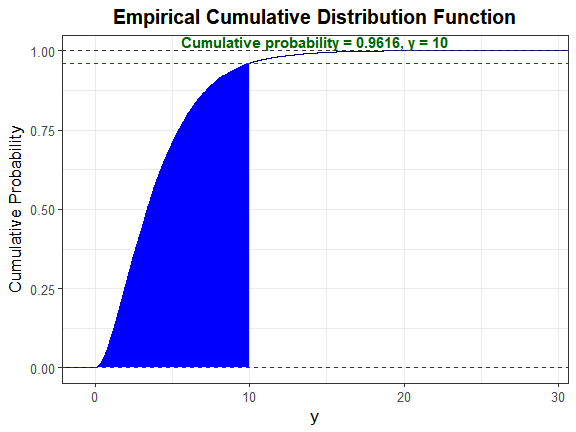1. Simulated Data and Target Distribution PDFs
plot_sim_pdf_theory(B$continuous_variables[, 2], Dist = "Chisq", params = 4)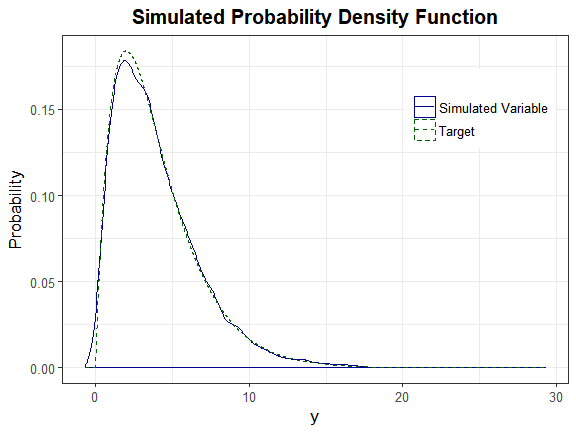Look at the empirical cdf of the $$\Large 2^{nd}$$ ordinal distribution: plot_sim_cdf(B$ordinal_variables[, 2])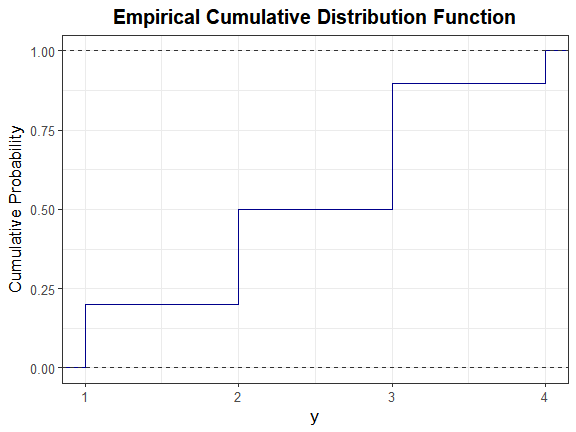Look at the Poisson ($$\Large \lambda = 5$$) distribution ($$\Large 2^{nd}$$ Poisson variable):

1. Simulated Data Values and Target Distribution
plot_sim_theory(B$Poisson_variables[, 2], cont_var = FALSE, Dist = "Poisson", params = 5)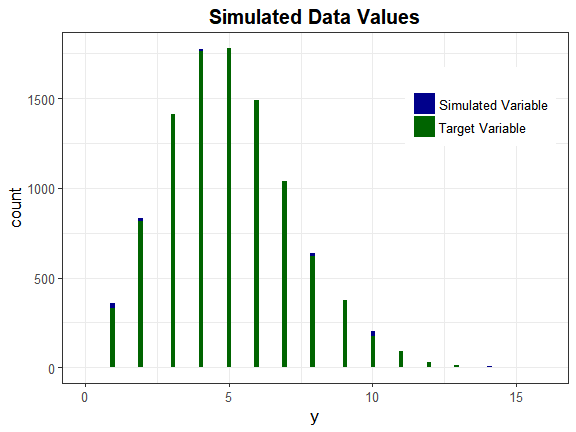1. Simulated Data and Target Distribution PDFs plot_sim_pdf_theory(B$Poisson_variables[, 2], cont_var = FALSE,
Dist = "Poisson", params = 5)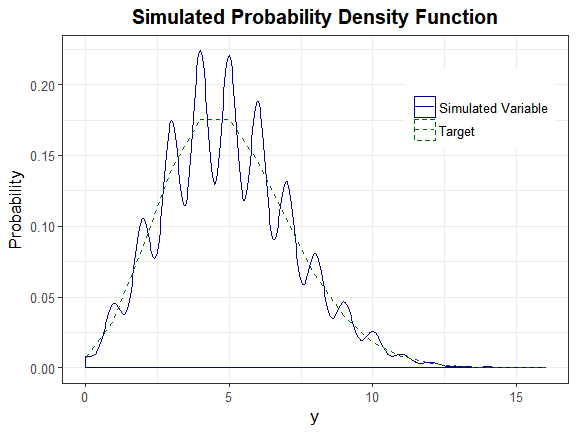Look at the Negative Binomial ($$\Large size = 3,\ prob = 0.2$$) distribution ($$\Large 1^{st}$$ Negative Binomial variable):

1. Simulated Data Values and Target Distribution
plot_sim_theory(B$Neg_Bin_variables[, 1], cont_var = FALSE, Dist = "Negative_Binomial", params = c(3, 0.2))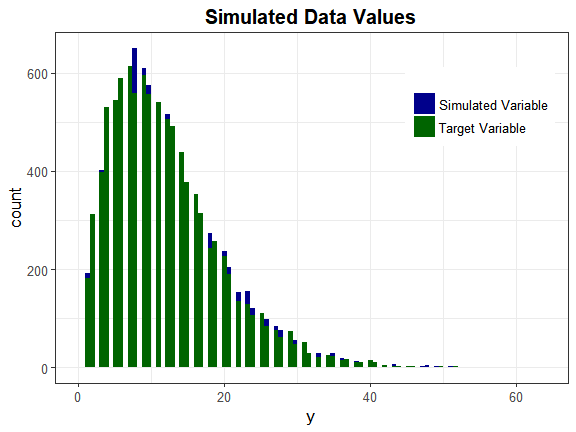1. Simulated Data and Target Distribution PDFs plot_sim_pdf_theory(B$Neg_Bin_variables[, 1], cont_var = FALSE,
Dist = "Negative_Binomial", params = c(3, 0.2))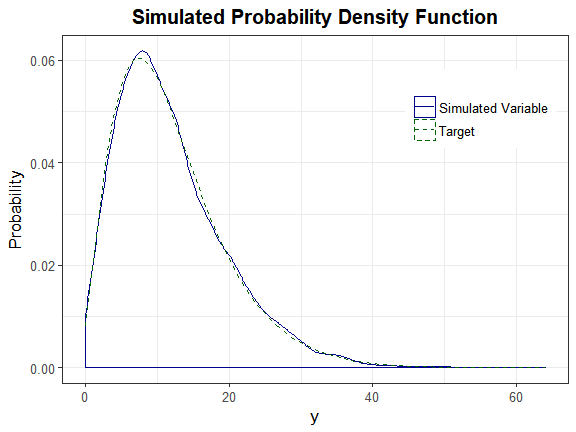### Correlation Method 2

Method 2 requires cumulative probability truncation vectors for the count variables (pois_eps for Poisson and nb_eps for Negative Binomial). Each entry is the amount removed from the total cumulative probability when creating a finite support for that variable (see max_count_support). The entries may vary by variable.

#### Step 3: Verify the target correlation matrix falls within the feasible correlation bounds

pois_eps <- rep(0.0001, npois)
nb_eps <- rep(0.0001, nnb)

# Make sure Rey is within upper and lower correlation limits
valid2 <- valid_corr2(k_cat = ncat, k_cont = ncont, k_pois = npois,
k_nb = nnb, method = "Polynomial", means =  M[1, ],
vars =  (M[2, ])^2, skews = M[3, ], skurts = M[4, ],
fifths = M[5, ], sixths = M[6, ], marginal = marginal,
lam = lam, pois_eps = pois_eps, size = size,
prob = prob, nb_eps = nb_eps, rho = Rey, seed = seed)
##
##  Constants: Distribution  1
##
##  Constants: Distribution  2
##
##  Constants: Distribution  3
## All correlations are in feasible range!

#### Step 4: Generate the variables

Simulate variables without the error loop.

C <- rcorrvar2(n = 10000, k_cont = ncont, k_cat = ncat, k_pois = npois,
k_nb = nnb, method = "Polynomial", means =  M[1, ],
vars =  (M[2, ])^2, skews = M[3, ], skurts = M[4, ],
fifths = M[5, ], sixths = M[6, ], marginal = marginal,
lam = lam, pois_eps = pois_eps, size = size, prob = prob,
nb_eps = nb_eps, rho = Rey, seed = seed)
##
##  Constants: Distribution  1
##
##  Constants: Distribution  2
##
##  Constants: Distribution  3
##
## Constants calculation time: 0 minutes
## Intercorrelation calculation time: 0.044 minutes
## Error loop calculation time: 0 minutes
## Total Simulation time: 0.046 minutes

Summarize the correlation errors:

Ccorr_error = round(C$correlations - Rey, 6) summary(as.numeric(Ccorr_error)) Min. 1st Qu. Median Mean 3rd Qu. Max. -0.014747 -0.000208 0.00084 0.0033461 0.005279 0.050601 These results indicate that for these distributions, Correlation Method 1 and Correlation Method 2 have similar correlation errors. Simulate variables with the error loop (using default settings of epsilon = 0.001 and maxit = 1000). D <- rcorrvar2(n = 10000, k_cont = ncont, k_cat = ncat, k_pois = npois, k_nb = nnb, method = "Polynomial", means = M[1, ], vars = (M[2, ])^2, skews = M[3, ], skurts = M[4, ], fifths = M[5, ], sixths = M[6, ], marginal = marginal, lam = lam, pois_eps = pois_eps, size = size, prob = prob, nb_eps = nb_eps, rho = Rey, seed = seed, errorloop = TRUE) ## ## Constants: Distribution 1 ## ## Constants: Distribution 2 ## ## Constants: Distribution 3 ## ## Constants calculation time: 0 minutes ## Intercorrelation calculation time: 0.045 minutes ## Error loop calculation time: 0.145 minutes ## Total Simulation time: 0.191 minutes Summarize the correlation errors: Dcorr_error = round(D$correlations - Rey, 6)
summary(as.numeric(Dcorr_error))
Min. 1st Qu. Median Mean 3rd Qu. Max.
-0.008774 -0.002094 -0.0004585 -0.0009118 0.000423 0.004498

Based on the interquartile range, the simulation utilizing the error loop will be chosen for subsequent analysis.

#### Step 5: Summarize the results numerically

1) Ordinal variables

knitr::kable(D$summary_ordinal[], caption = "Variable 1") Variable 1 Target Simulated 0.30 0.2965 0.75 0.7500 1.00 1.0000 knitr::kable(D$summary_ordinal[], caption = "Variable 2")
Variable 2
Target Simulated
0.2 0.1997
0.5 0.4984
0.9 0.8976
1.0 1.0000

2) Count variables

Poisson variables: Note the expected means and variances are also given.

as.matrix(D$summary_Poisson[, c(1, 3:6, 8:9)], nrow = 3, ncol = 7, byrow = TRUE) Distribution mean Exp_mean var Exp_var min max 1 0.9936 1 1.006460 1 0 8 2 5.0006 5 4.993499 5 0 16 3 10.0018 10 9.995996 10 1 28 Negative Binomial variables: as.matrix(D$summary_Neg_Bin[, c(1, 3:7, 9:10)], nrow = 2, ncol = 8,
byrow = TRUE)
Distribution success_prob mean Exp_mean var Exp_var min max
1 0.2 11.9947 12.0 59.959268 60.000 0 65
2 0.8 1.5030 1.5 1.876979 1.875 0 9

3) Continuous variables

The constants are the same for both methods.

Target distributions:

as.matrix(round(D$summary_targetcont, 5), nrow = 3, ncol = 7, byrow = TRUE) Distribution mean sd skew skurtosis fifth sixth M1 1 0.00000 1.00000 0.00000 0.000 0.00000 0.00000 M2 2 4.00000 2.82843 1.41421 3.000 8.48528 30.00000 M3 3 0.66667 0.17817 -0.46771 -0.375 1.40312 -0.42614 Simulated distributions: as.matrix(round(D$summary_continuous[, c("Distribution", "mean", "sd",
"skew", "skurtosis", "fifth",
"sixth")], 5), nrow = 3, ncol = 7,
byrow = TRUE)
Distribution mean sd skew skurtosis fifth sixth
X1 1 0.00000 1.00000 0.04377 -0.00771 0.14892 0.63578
X2 2 3.99846 2.81011 1.37595 2.84004 8.40445 37.70069
X3 3 0.66643 0.17767 -0.43926 -0.40314 1.27686 -0.02855

Valid power method pdf check:

D$valid.pdf ##  "TRUE" "TRUE" "TRUE" All continuous variables have valid power method pdfs. We can compute additional summary statistics: 1) Normal($$\Large 0,1$$) Distribution as.matrix(t(round(stats_pdf(c = D$constants[1, ], method = "Polynomial",
alpha = 0.025), 4)))
trimmed_mean median mode max_height
0 0 0 0.3989
1. Chisq ($$\Large df = 4$$) Distribution
as.matrix(t(round(stats_pdf(c = B$constants[2, ], method = "Polynomial", alpha = 0.025), 4))) trimmed_mean median mode max_height -0.0536 -0.2275 -0.7095 0.5203 1. Beta ($$\Large \alpha = 4, \beta = 2$$) Distribution as.matrix(t(round(stats_pdf(c = B$constants[3, ], method = "Polynomial",
alpha = 0.025), 4)))
trimmed_mean median mode max_height
0.0215 0.1083 0.4517 0.3745

#### Step 6: Summarize the results graphically

Since the methods vary primarily according to the calculation of the intermediate correlation for count variables, we will only look at the distributions of two count variables.

Look at the Poisson ($$\Large \lambda = 5$$) distribution ($$\Large 2^{nd}$$ Poisson variable):

1. Simulated Data Values and Target Distribution
plot_sim_theory(D$Poisson_variables[, 2], cont_var = FALSE, Dist = "Poisson", params = 5)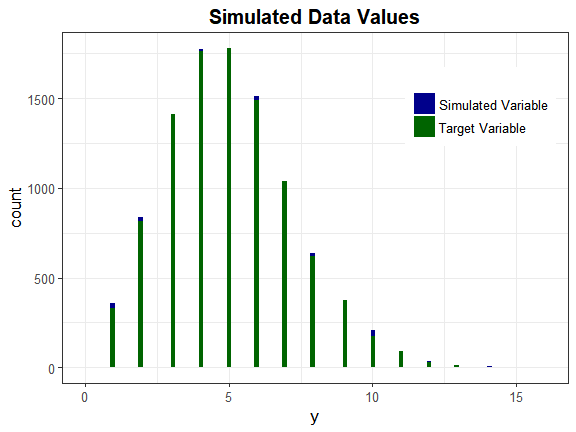1. Simulated Data and Target Distribution PDFs plot_sim_pdf_theory(D$Poisson_variables[, 2], cont_var = FALSE,
Dist = "Poisson", params = 5)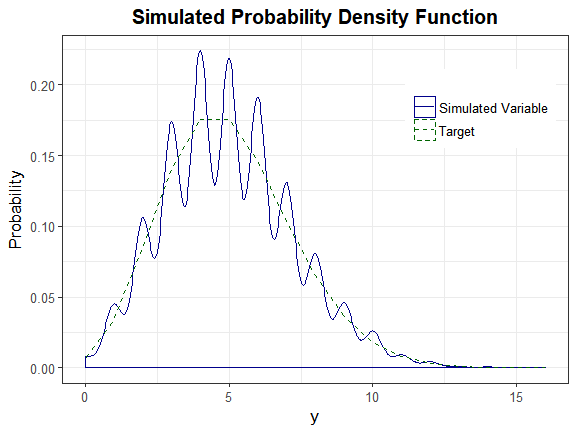Look at the Negative Binomial ($$\Large size = 3,\ prob = 0.2$$) distribution ($$\Large 1^{st}$$ Negative Binomial variable):

1. Simulated Data Values and Target Distribution
plot_sim_theory(D$Neg_Bin_variables[, 1], cont_var = FALSE, Dist = "Negative_Binomial", params = c(3, 0.2))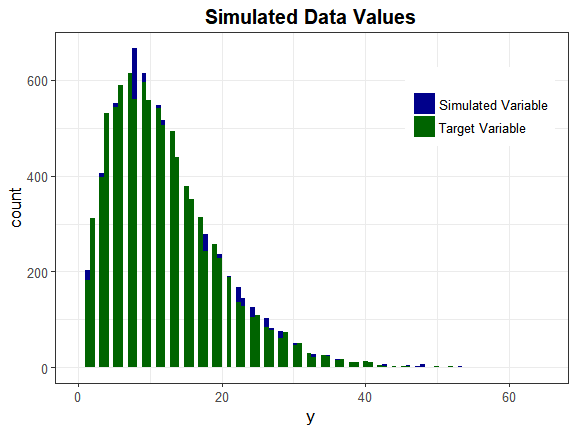1. Simulated Data and Target Distribution PDFs plot_sim_pdf_theory(D$Neg_Bin_variables[, 1], cont_var = FALSE,
Dist = "Negative_Binomial", params = c(3, 0.2))